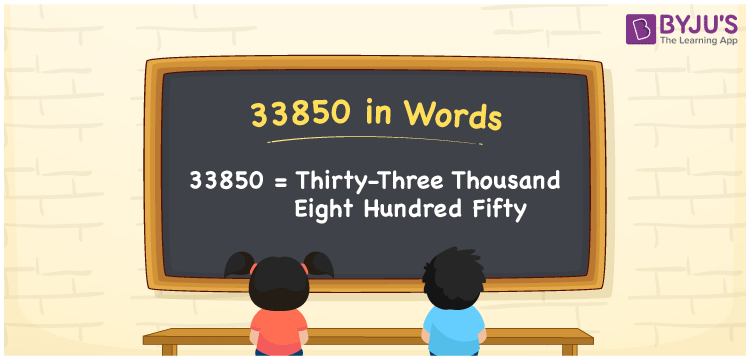# 33850 in Words

33850 in words is written as Thirty-three thousand eight hundred fifty. In both the International System of Numerals and the Indian System of Numerals, 33850 is written as Thirty-three thousand eight hundred fifty. The number 33850 is a Cardinal Number as it represents some quantity. For example, “the population of this village is about 33850”.

 33850 in Words Thirty-three thousand eight hundred fifty Thirty-three thousand eight hundred fifty in Number 33850

## 33850 in English Words

33850 in English words is read as “Thirty-three thousand eight hundred fifty”.## How to Write 33850 in Words?

To write 33850 in words, we shall use the place value chart. In the place value chart, put 3 in the ten thousands and thousands, 8 in the hundreds, 5 in the tens and 0 in the ones. Let us make a place value chart to write the number 33850 in words.

 Ten Thousands Thousands Hundreds Tens Ones 3 3 8 5 0

Thus, we can write the expanded form as

3 × Ten Thousand + 3 × Thousand + 8 × Hundred + 5 × Ten + 0 × One

= 3 × 10000 + 3 × 1000 + 8 × 100 + 5 × 10 + 0 × 1

= 30000 + 3000 + 800 +50 + 0

= 33850

= Thirty-three thousand eight hundred fifty.

33850 is a natural number, the successor of 33849 and the predecessor of 33851.

33850 in words – Thirty-three thousand eight hundred fifty

• Is 33850 an odd number? – No
• Is 33850 an even number? – Yes
• Is 33850 a perfect square number? – No
• Is 33850 a perfect cube number? – No
• Is 33850 a prime number? – No
• Is 33850 a composite number? – Yes

## Frequently Asked Questions on 33850 in Words

Q1

### How to write 33850 in words?

33850 in words is written as Thirty-three thousand eight hundred fifty.
Q2

### How to write 33850 in the International and Indian System of Numerals?

In both, the system of numerals, 33850 in words, is written as Thirty-three thousand eight hundred fifty.
Q3

### How to write 33850 in a place value chart?

In the place value chart, write 3 in the ten thousands and thousands, 8 in the hundreds, 5 in the tens and 0 in the ones, respectively.